# Test Prep Plan - Take a practice test

Take this practice test to check your existing knowledge of the course material. We'll review your answers and create a Test Prep Plan for you based on your results.
How Test Prep Plans work
1
2Based on your results, we'll create a customized Test Prep Plan just for you!
3Study smarter
Study more effectively: skip concepts you already know and focus on what you still need to learn.

# MTEL Mathematics (Elementary): Properties of Triangles Chapter Exam

Exam Instructions:

Choose your answers to the questions and click 'Next' to see the next set of questions. You can skip questions if you would like and come back to them later with the yellow "Go To First Skipped Question" button. When you have completed the practice exam, a green submit button will appear. Click it to see your results. Good luck!

### Page 1

#### Question 1 1. Mary claims that the triangles below are similar by AA. Do you agree or disagree with Mary?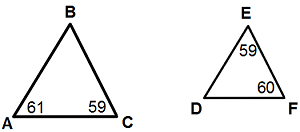#### Question 2 2. In the figure below, Angle 13 = 10y and Angle 11 = 3y + 11. Determine the measure of Angle 16.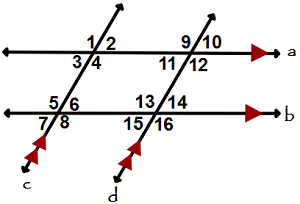#### Question 3 3. If angle a is 30 degrees and angle d is 70 degrees, what is the measure of angle b?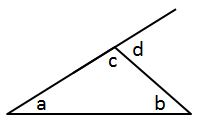#### Question 4 4. Given the triangle ABC, solve for the value of y.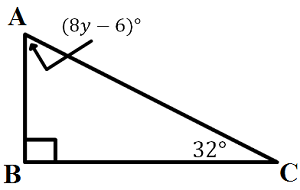### Page 2

#### Question 6 6. On a map, B St., Smith Rd., and Powell St. form a triangle. Jones Way forms the midsegment of this triangle. The perimeter of the triangle formed by B St., Smith Rd., and Powell St. is 4 inches long. What is the perimeter of the triangle formed by B. St., Smith Rd. and Jones Way?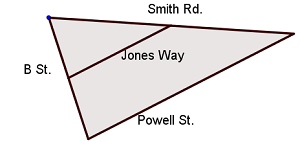#### Question 8 8. What is the area of the pictured rectangle?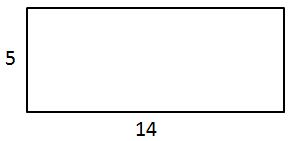#### Question 9 9. Conclude whether triangle BEN is similar to triangle MAT.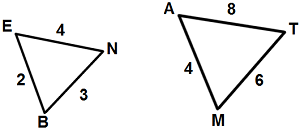#### Question 10 10. A surveyor is measuring the width of a pond. She chooses a landmark on the opposite side of the pond, and measures the angle to this landmark from a point 50 ft away from the original point. How wide is the pond (x)?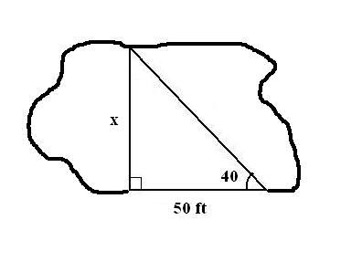### Page 3

#### Question 11 11. In the picture below, segment DE is a midsegment of triangle ABC. If DE is parallel to AC and DE has a length of 10 feet, how long is AC?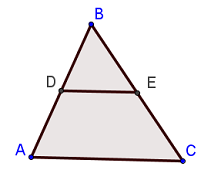#### Question 12 12. Josh claims that these triangles are similar by SSS. Do you agree or disagree with Josh?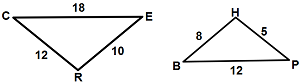#### Question 13 13. What type of triangle is pictured?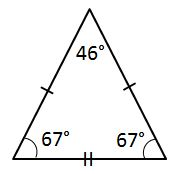#### Question 15 15. What type of triangle is pictured?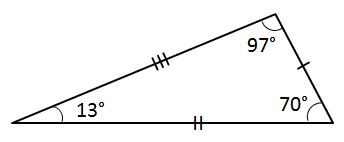### Page 4

#### Question 16 16. In triangle GBN, Angle G is seven more than three times a number, Angle B is five more than four times the number, and Angle N is twelve less than five times the number. Solve for the measure of Angle G.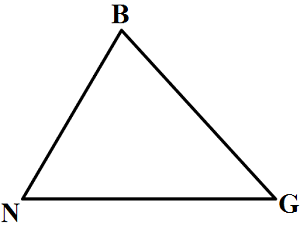#### Question 17 17. If angle a is 68 degrees and angle b is 37 degrees, what is the measure of angle c?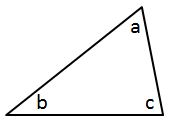#### Question 20 20. Is triangle ABC similar to triangle DEF?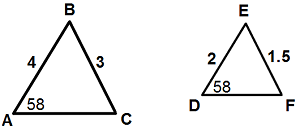### Page 5

#### Question 21 21. If angle a is 66 degrees and angle b is 54 degrees, what is the measure of angle d?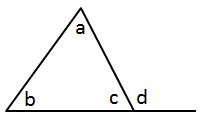#### Question 22 22. In the picture below, segment DE is a midsegment of triangle ABC. If DE is parallel to AC and AC has a length of 30 feet, how long is DE?#### Question 23 23. Using the Pythagorean theorem method, find the height of the following triangle.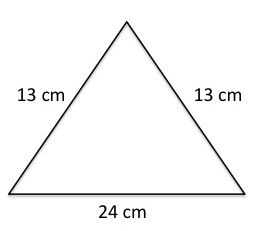### Page 6

#### Question 26 26. If angle a is 80 degrees, what is the measure of angle d?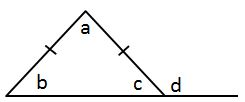#### Question 27 27. In the pictured triangle, what is side B, relative to angle x?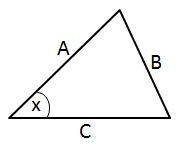#### Question 28 28. What is the area of ABCDE, as shown in the diagram?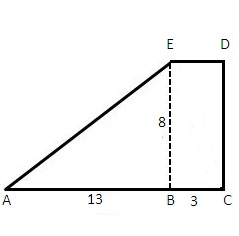#### Question 29 29. What is the distance from A to B?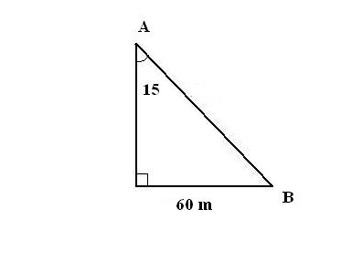#### Question 30 30. In the picture below, segment DE is a midsegment of triangle ABC. Which of the following statements is NOT always true?#### MTEL Mathematics (Elementary): Properties of Triangles Chapter Exam Instructions

Choose your answers to the questions and click 'Next' to see the next set of questions. You can skip questions if you would like and come back to them later with the yellow "Go To First Skipped Question" button. When you have completed the practice exam, a green submit button will appear. Click it to see your results. Good luck!

Support